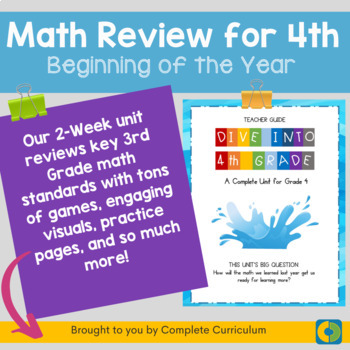# 4th Grade Beginning of the Year Math Review: 2021 Edition3rd - 4th
Subjects
Standards
Resource Type
Formats Included
•Google Drive™ folder
Pages
98 pages### Description

This two-week unit comes with printables, online student visual support, comprehensive lesson plans, and engaging activities that will help your fourth graders start the year with a math-packed review of third grade standards. Most practice pages come in both printable and self-graded Google Form formats just in case you have to do something remotely. It is also a fantastic set of lessons to use for intervention!

Teaching Remotely? Check out the Distance Learning Edition of this Unit!

Do you have a student who needs more practice? Check out our 6-Week Unit with in-depth, independent review lessons.

More from Complete Curriculum here!

This unit was designed as a beginning-of-the-year review for incoming fourth-grade students. It addresses these critical focus areas in math for third grade:

Understanding multiplication and division strategies:

• Representing and solving problems involving multiplication and division.

• Understanding properties of multiplication and the relationship between multiplication and division.

• Multiplying and dividing within 100.

Developing an understanding of fractions:

• Using fractions along with visual models to represent parts of a whole, relative to the size of the whole

• Solving problems that involve comparing fractions

Understanding area:

• Measuring the area of a shape by finding the total number of units

• Composing and decomposing rectangular arrays and connect it to multiplication

Describing and analyzing 2D shapes:

• Comparing and classifying shapes by their sides and angles

• Expressing parts of shapes as unit fractions of the whole

Total Pages
98 pages
Included
Teaching Duration
2 Weeks
Report this Resource to TpT
Reported resources will be reviewed by our team. Report this resource to let us know if this resource violates TpT’s content guidelines.

### Standards

to see state-specific standards (only available in the US).
Interpret products of whole numbers, e.g., interpret 5 Ã 7 as the total number of objects in 5 groups of 7 objects each. For example, describe a context in which a total number of objects can be expressed as 5 Ã 7.
Interpret whole-number quotients of whole numbers, e.g., interpret 56 Ã· 8 as the number of objects in each share when 56 objects are partitioned equally into 8 shares, or as a number of shares when 56 objects are partitioned into equal shares of 8 objects each. For example, describe a context in which a number of shares or a number of groups can be expressed as 56 Ã· 8.
Use multiplication and division within 100 to solve word problems in situations involving equal groups, arrays, and measurement quantities, e.g., by using drawings and equations with a symbol for the unknown number to represent the problem.
Fluently multiply and divide within 100, using strategies such as the relationship between multiplication and division (e.g., knowing that 8 Ã 5 = 40, one knows 40 Ã· 5 = 8) or properties of operations. By the end of Grade 3, know from memory all products of two one-digit numbers.
Understand a fraction as a number on the number line; represent fractions on a number line diagram.